# Best Quantum Computing Books 2023 | Learn Quantum Computing

Quantum computing applies the properties of quantum mechanics such as superposition and entanglement to process information. The computation must be implemented theoretically or physically.

In the following, you will get some Best quantum computing books in 2023

## 1. Quantum Computing Fundamentals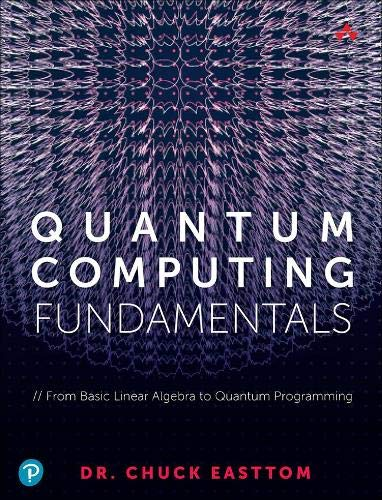Author: Easttom II, William (Chuck)
Published at: 01/06/2021
ISBN: 0136793819

Whether you’re a student, professional, or manager, this guide will prepare you for the quantum computing revolution--and expand your career options, too.

• Master the linear algebra and other mathematical skills you’ll need
• Explore key physics ideas such as quantum states and uncertainty
• Review data structures, algorithms, and computing complexity
• Work with probability and set theory in quantum computing
• Familiarize yourself with basic quantum theory and formulae
• Understand quantum entanglement and quantum key distribution
• Discover how quantum computers are architected and built
• Explore several leading quantum algorithms
• Compare quantum and conventional asymmetric algorithms
• See how quantum computing might break traditional cryptography
• Discover several approaches to quantum-resistant cryptography
• Start coding with Q#, Microsoft’s quantum programming language
• Simulate quantum gates and algorithms with QASM

## 2. Quantum physics for beginners: From Wave Theory to Quantum Computing. Understanding How Everything Works by a Simplified Explanation of Quantum Physics and Mechanics Principles

In this book you will find:

• What quantum physics is, the history and most famous experiments and achievements in quantum mechanics.
• Wave-particle duality dilemma.
• Heisenberg uncertainty principle.
• Schrodinger's equation.
• Quantum fields theory.
• Introduction to string theory.
• Real-world applications: Quantum computing, Quantum key distribution...

## 3. Learn Quantum Computing with Python and IBM Quantum Experience: A hands-on introduction to quantum computing and writing your own quantum programs with Python

#### What you will learn in this quantum computing book:

• Explore quantum computational principles such as superposition and quantum entanglement
• Become familiar with the contents and layout of the IBM Quantum Experience
• Understand quantum gates and how they operate on qubits
• Discover the quantum information science kit and its elements such as Terra and Aer
• Get to grips with quantum algorithms such as Bell State, Deutsch-Jozsa, Grover's algorithm, and Shor's algorithm
• How to create and visualize a quantum circuit

## 4. Mathematics of Quantum Computing: An Introduction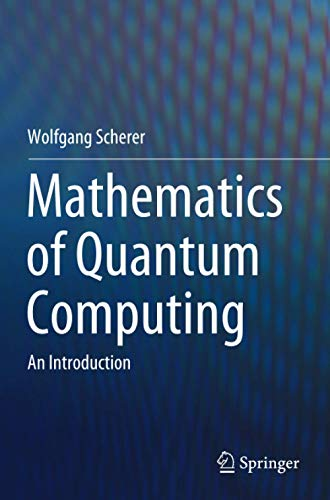Author: Scherer, Wolfgang
Published at: 22/11/2020
ISBN: 303012360X

This textbook presents the elementary aspects of quantum computing in a mathematical form. It is intended as core or supplementary reading for physicists, mathematicians, and computer scientists taking the first course on quantum computing. It starts by introducing the basic mathematics required for quantum mechanics and then goes on to present, in detail, the notions of quantum mechanics, entanglement, quantum gates, and quantum algorithms, of which Shor's factorisation and Grover's search algorithm are discussed extensively.

In addition, the algorithms for the Abelian Hidden Subgroup and Discrete Logarithm problems are presented and the latter is used to show how the Bitcoin digital signature may be compromised. It also addresses the problem of error correction as well as giving a detailed exposition of adiabatic quantum computing. The book contains around 140 exercises for the student, covering all of the topics treated, together with an appendix of solutions.

## 5. Quantum Computing for Everyone (The MIT Press)

If you want to jump into the world of quantum computing then Quantum Computing for Everyone is for you. This book is very clear to understand and is an entry-level book. Prior knowledge of linear algebra is required if anyone wants to understand the basics of how and why a quantum computer works and some of the concepts of Quantum Mechanics.

In this book, Chris Bernhardt explained

• An introduction to quantum computing
• The basic unit of quantum computing
• How the qubit can be measured
• Qubits, entanglement, quantum teleportation
• Quantum gates
• The speed of quantum algorithms
• The building of quantum computers
• Quantum algorithms.

## 6. Quantum Computing: A Gentle Introduction (Scientific and Engineering Computation)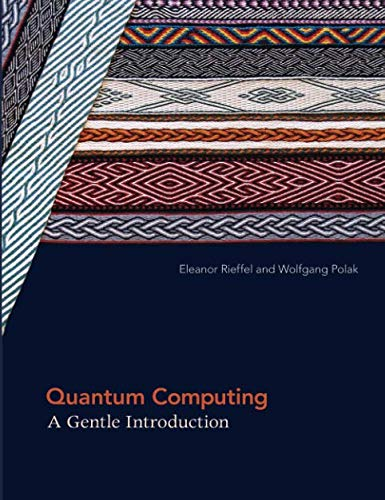Author: Eleanor G. Rieffel,Wolfgang H. Polak
Published at: 29/08/2014
ISBN: 0262526670

Quantum Computing: A Gentle Introduction is an excellent book containing exercises which are useful, explanations are well thought out and written. Full of information, examples, and formulas. This quantum computing books also contains the necessary information to understand programming.

This book provides you the combination of two of the twentieth century's most influential topics like

• Revolutionary scientific theories
• Information theory and
• Quantum mechanics

It offers you a thorough exposition of quantum computing and the underlying concepts of quantum physics, explaining all the relevant mathematics and offering numerous examples. Students and professionals in mathematics, computer science, and engineering can access to the quantum computing of this book.

## 7. Quantum Computing since Democritus

People having some knowledge in math like complex numbers, probability, and matrices (linear algebra), physics and computer science will find Quantum Computing since Democritus very beneficial. Those who are interested in philosophy, life, and the universe will definitely enjoy the best quantum computing books.

This book offers you

• Logic and set theory
• Computability and complexity theory
• Quantum computing
• Cryptography
• The information content of quantum states
• The interpretation of quantum mechanics
• Time travel,
• The anthropic principle
• The views of Roger Penrose.

## 8. Quantum Computing for Babies (Baby University)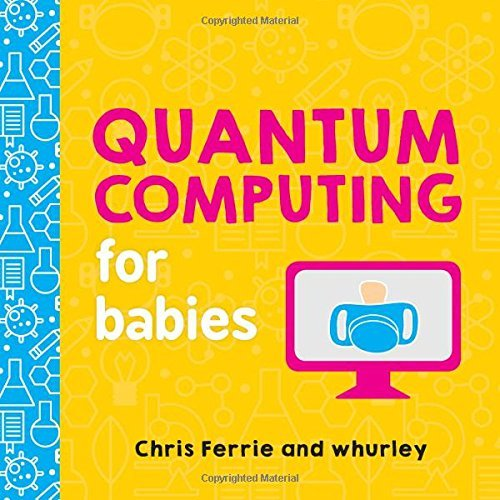Author: Chris Ferrie,whurley
Published at: 03/04/2018
ISBN: 1492671185

Quantum Computing for Babies provides a simple and straightforward explanation. It offers your younger daughter or sister a great way to learn quantum computing. All the complex ideas are explained simply.

You'll discover

• The difference between bits and qubits
• How quantum computers will change our future
• Bigger vocabulary and exposure to complex concepts.

## 9. Mastering Quantum Computing with IBM QX: Explore the world of quantum computing using the Quantum Composer and Qiskit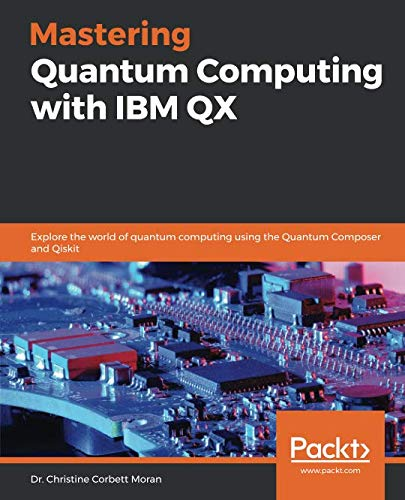Author: Dr. Christine Corbett Moran
Published at: 31/01/2019
ISBN: 1789136431

This book provides you the core concepts and principles of quantum computing. You will know the area where quantum principles can be applied. Again you'll be able to design programs with quantum logic. This book shows you the way of developing the ability to analyze the potential of quantum computing in your industry.

Key topics are

• What is quantum computing?
• Qubits
• Quantum states, quantum registers, and measurement
• Quantum gates
• Quantum circuits
• The Quantum composer
• Working with OpenQASM 2.0
• QISKit and quantum computer simulation
• Quantum AND (Toffoli) gates and quantum OR gates
• Grover's algorithm
• Quantum Fourier transform
• Shor's algorithm
• Quantum error correction
• The future of quantum computing.

## 10. Quantum Computation and Quantum Information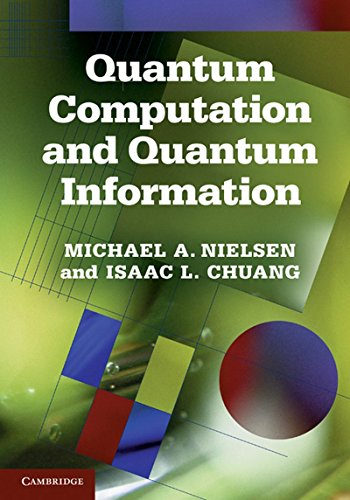Author: Michael A. Nielsen Isaac L. Chuang
Published at: 01/01/2013
ISBN: 110761919X

Quantum Computation and Quantum Information is a perfect study guide for those interested in modern Quantum physics. This is a solid reference material for a complex subject.

Key contents include

• Introduction and overview
• Introduction to quantum mechanics
• Introduction to computer science
• Quantum circuits
• The quantum Fourier transform and its applications
• Quantum search algorithms
• Quantum computers: physical realization
• Quantum noise and quantum operations
• Distance measures for quantum information
• Quantum error-correction
• Entropy and information
• Quantum information theory.

## 11. Practical Quantum Computing for Developers: Programming Quantum Rigs in the Cloud using Python, Quantum Assembly Language and IBM QExperience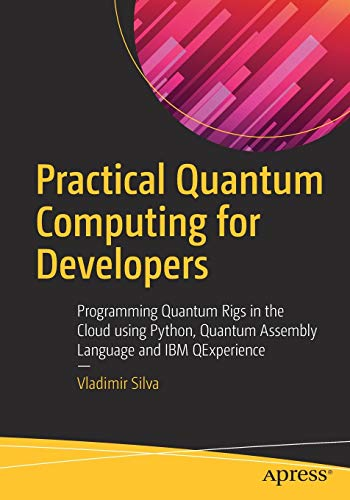Published at: 13/12/2018
ISBN: 1484242173

Also, Practical Quantum Computing for Developers discusses quantum computing in the cloud using IBM Q Experience including the composer, quantum scores, experiments, circuits, simulators, real quantum devices, and more. You’ll be able to run experiments in the cloud on a real quantum device.

You will get:

• Use the Q Experience Composer, the first-of-its-kind web console to create visual programs/experiments and submit them to a quantum simulator or real device on the cloud.
• Run programs remotely using the Q Experience REST API.
• Write algorithms that provide superior performance over their classical counterparts.
• Build a Node.js REST client for authenticating, listing remote devices, querying information about quantum processors, and listing or running experiments remotely in the cloud.
• Create a quantum number generator: The quintessential coin flip with a quantum twist.
• Discover quantum teleportation: This algorithm demonstrates how the exact state of a qubit (quantum information) can be transmitted from one location to another, with the help of classical communication and quantum entanglement between the sender and receiver.
• Peek into single-qubit operations with the classic game of Battleships with a quantum twist.
• Handle the counterfeit coin problem: a classic puzzle that consists of finding a counterfeit coin in a beam balance among eight coins in only two turns.

## 12. Programming Quantum Computers: Essential Algorithms and Code Samples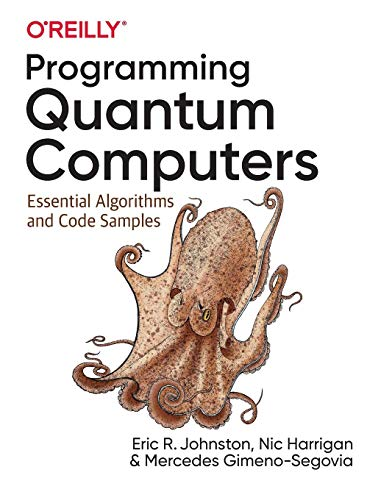Author: Johnston, Eric R.
Published at: 30/07/2019
ISBN: 1492039683

This book includes three multichapter sections:

• Programming for a QPU—Explore core concepts for programming quantum processing units and include how to describe and manipulate qubits and how to perform quantum teleportation.
• QPU Primitives—Learn algorithmic primitives and techniques, including amplitude amplification, the Quantum Fourier Transform, and phase estimation.
• QPU Applications—Investigate how QPU primitives are used to building existing applications, including quantum search techniques and Shor’s factoring algorithm.

## 13. Dancing with Qubits: How quantum computing works and how it can change the world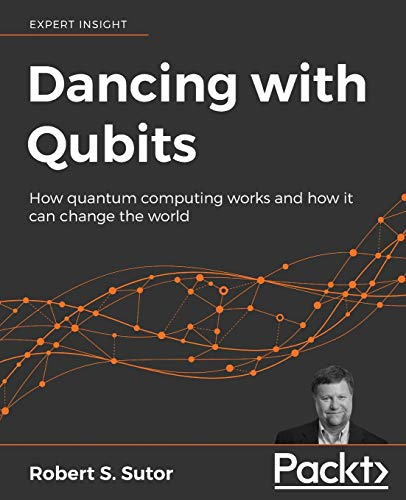Author: Sutor, Robert S.
Published at: 28/11/2019
ISBN: 1838827366

#### What you will learn

• See how quantum computing works, delve into the math behind it, what makes it different, and why it is so powerful with this quantum computing textbook
• Discover the complex, mind-bending mechanics that underpin quantum systems
• Understand the necessary concepts behind classical and quantum computing
• Refresh and extend your grasp of essential mathematics, computing, and quantum theory
• Explore the main applications of quantum computing to the fields of scientific computing, AI, and elsewhere
• Examine a detailed overview of qubits, quantum circuits, and quantum algorithm

## 14. Quantum Computing: An Applied Approach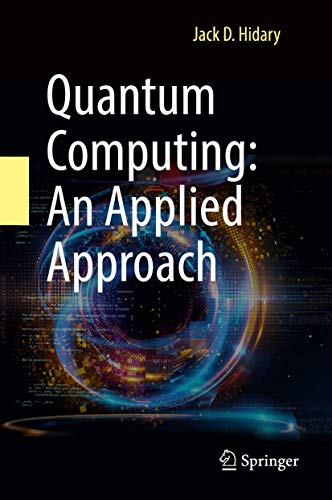Author: Hidary, Jack D.
Published at: 20/09/2019
ISBN: 3030239217

This volume comprises three books under one cover: Part I outlines the necessary foundations of quantum computing and quantum circuits. Part II walks through the canon of quantum computing algorithms and provides code on a range of quantum computing methods in current use. Part III covers the mathematical toolkit required to master quantum computing. Additional resources include a table of operators and circuit elements and a companion GitHub site providing code and updates.

Jack D. Hidary is a research scientist in quantum computing and in AI at Alphabet X, formerly Google X.

“Quantum Computing will change our world in unexpected ways. Everything technology leaders, engineers and graduate students need is in this book including the methods and hands-on code to program on this novel platform.”

―Eric Schmidt, PhD, Former Chairman and CEO of Google; Founder, Innovation Endeavors.

## 15. Quantum Computation and Quantum Information: 10th Anniversary Edition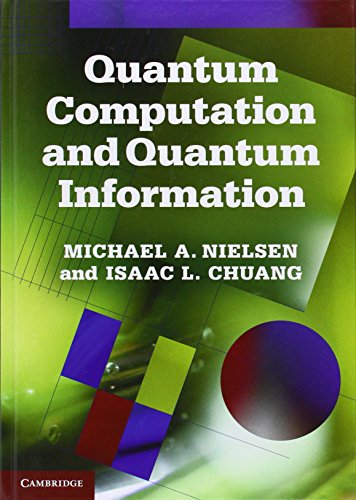Author: Nielsen, Michael A.
Published at: 31/01/2011
ISBN: 1107002176

Quantum Computation and Quantum Information remain the best textbook in this exciting field of science include an introduction from the authors setting the work in context. This comprehensive textbook describes such remarkable effects as fast quantum algorithms, quantum teleportation, quantum cryptography and quantum error correction.

Quantum mechanics and computer science are introduced before moving on to describe what a quantum computer is, how it can be used to solve problems faster than ‘classical' computers and its real-world implementation. It concludes with an in-depth treatment of quantum information. Containing a wealth of figures and exercises, this well-known textbook is ideal for courses on the subject and will interest beginning graduate students and researchers in physics, computer science, mathematics, and electrical engineering.

## 16. Quantum Mechanics: The Theoretical Minimum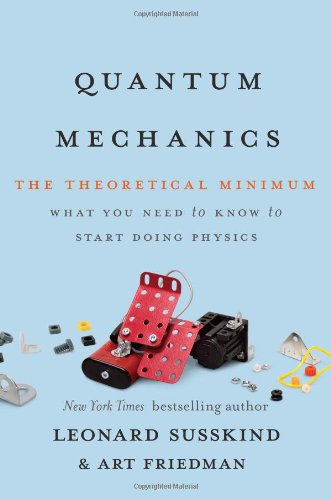Author: Susskind, Leonard
Published at: 25/02/2014
ISBN: 0465036678

First, he taught you, classical mechanics. Now, physicist Leonard Susskind has teamed up with data engineer Art Friedman to present the theory and associated mathematics of the strange world of quantum mechanics.

In this follow-up to the New York Times best-selling The Theoretical Minimum, Susskind and Friedman provide a lively introduction to this famously difficult field, which attempts to understand the behaviour of sub-atomic objects through mathematical abstractions. Unlike other popularizations that shy away from quantum mechanics' weirdness, Quantum Mechanics embraces the utter strangeness of quantum logic.

The authors offer crystal-clear explanations of the principles of quantum states, uncertainty and time dependence, entanglement, and particle and wave states, among other topics, and each chapter includes exercises to ensure mastery of each area. Like The Theoretical Minimum, this volume runs parallel to Susskind's eponymous Stanford University-hosted continuing education course.

An approachable yet rigorous introduction to a famously difficult topic, Quantum Mechanics provides a tool kit for amateur scientists to learn physics at their own pace.

## 17. Cryptography Apocalypse: Preparing for the Day When Quantum Computing Breaks Today's Crypto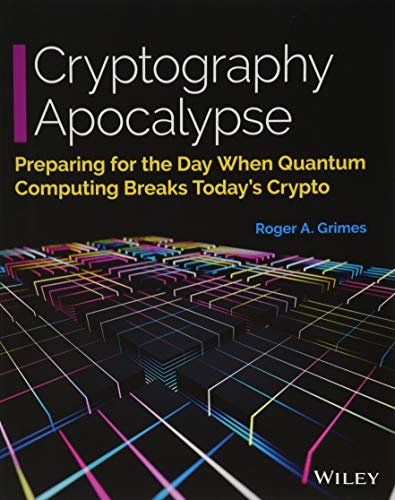Author: Grimes, Roger A.
Published at: 12/11/2019
ISBN: 1119618193

Cryptography Apocalypse is a crucial resource for every IT and InfoSec professional for preparing for the coming quantum-computing revolution. Post-quantum crypto algorithms are already a reality, but implementation will take significant time and computing power. This practical guide helps IT leaders and implementers make the appropriate decisions today to meet the challenges of tomorrow. This important book:

• Gives a simple quantum mechanics primer
• Explains how quantum computing will break current cryptography
• Offers practical advice for preparing for a post-quantum world
• Presents the latest information on new cryptographic methods
• Describes the appropriate steps leaders must take to implement existing solutions to guard against quantum-computer security threats

## 18. No-Nonsense Quantum Field Theory: A Student-Friendly Introduction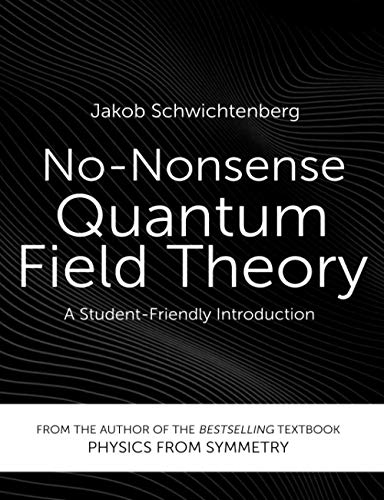Author: Schwichtenberg, Jakob
Published at: 25/02/2020
ISBN: 3948763011

What will you learn from this book?

• Get to know all fundamental concepts — Grasp what a quantum field is, why we use propagators to describe its behaviour, and how Feynman diagrams help us to make sense of field interactions.
• Learn to describe quantum field theory mathematically — Understand the meaning and origin of the most important equations: the Klein-Gordon equation, the Dirac equation, the Proca equation, the Maxwell equations, and the canonical commutation/anticommutation relations.
• Master important quantum field theory interactions — Read fully annotated, step-by-step calculations and understand the general algorithm we use for particle interactions.
• Get an understanding you can be proud of —Learn about advanced topics like renormalization and regularization, spontaneous symmetry breaking, the renormalization group equations, non-perturbative phenomena, and effective field models.

Thanks for reading this post. If you have any opinion don't hesitate to comment here. Also please subscribe our newsletter to get more updates.